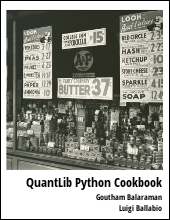This post will walk through some of the basics of QuantLib Python library.

Visit here for other QuantLib Python examples. If you found these posts useful, please take a minute by providing some feedback.

I installed the latest version of QuantLib (V1.5) and the python wrapper to QuantLib. My experiments lately have been to get a feel for the QuantLib API. The library itself is so extensive, that it is rather hard for a new comer to get going. In this post we will look into some of the basic classes and functionality in QuantLib.

Let us import QuantLib as:

import QuantLib as ql


## Time SubModule

The ql/time sub-folder implements various time related classes. Lets take a look at the Date object which can be constructed as Date(date, month, year).

>>> date = ql.Date(31, 3, 2015) # 31 March, 2015
>>> print date
March 31st, 2015
>>> date.dayOfMonth()
31
>>> date.month()
3
>>> date.year()
2015
>>> date.weekday() == ql.Tuesday
True

# arithmetic with dates
>>> date + 1  # add a day
Date(1,4,2015)
>>> date - 1  # subtract a day
Date(30,3,2015)
>>> date + ql.Period(1, ql.Months)
Date(30,4,2015)
>>> date + ql.Period(1, ql.Weeks)
Date(7,4,2015)
>>> date + ql.Period(1, ql.Years)
Date(31,3,2016)

# logical operations
>>> ql.Date(31, 3, 2015) > ql.Date(1, 3, 2015)
True


The Schedule object can be used to construct a list of dates such as coupon payments. Lets look at some examples.

>>> date1 = ql.Date(1, 1, 2015)
>>> date2 = ql.Date(1, 1, 2016)
>>> tenor = ql.Period(ql.Monthly)
>>> calendar = ql.UnitedStates()
>>>
>>> schedule = ql.Schedule(date1, date2, tenor, calendar, ql.Following,
ql.Following, ql.DateGeneration.Forward, False)
>>> list(schedule)
[Date(2,1,2015),
Date(2,2,2015),
Date(2,3,2015),
Date(1,4,2015),
Date(1,5,2015),
Date(1,6,2015),
Date(1,7,2015),
Date(3,8,2015),
Date(1,9,2015),
Date(1,10,2015),
Date(2,11,2015),
Date(1,12,2015),
Date(4,1,2016)]


Here we have generated a Schedule object that will contain dates between date1 and date2 with the tenor specifying the Period to be every Month. The calendar object is used for determining holidays. The two arguments following the calendar in the Schedule constructor are the BussinessDayConvention. Here we chose the convention to be the day following holidays. That is why we see that holidays are excluded in the list of dates.

## Interest Rate

The InterestRate class can be used to store the interest rate with the compounding type, day count and the frequency of compounding. Below we show how to create an interest rate of 5.0% compounded annually, using Actual/Actual day count convention.

>>> annualRate = 0.05
>>> dayCount = ql.ActualActual()
>>> compoundType = ql.Compounded
>>> frequency = ql.Annual
>>> interestRate = ql.InterestRate(annualRate, dayCount, compoundType, frequency)


Lets say if you invest a dollar at the interest rate described by interestRate, the compoundFactor method gives you how much your investment will be worth after t years. Below we show that the value returned by compoundFactor for 2 years agrees with the expected compounding formula.

>>> interestRate.compoundFactor(2.0)
1.1025
>>> (1.0 + annualRate)*(1.0 + annualRate)  # Check the above calculation
1.1025


The discountFactor method returns the reciprocal of the compoundFactor method. The discount factor is useful while calculating the present value of future cashflows.

>>> interestRate.discountFactor(2.0)
0.9070294784580498
>>> 1.0 / interestRate.compoundFactor(2.0)
0.9070294784580498


A given interest rate can be converted into other types using the equivalentRate method as :

>>> newFrequency = ql.Semiannual
>>> effectiveRate = interestRate.equivalentRate(compoundType, newFrequency, 1)
>>> effectiveRate.rate()
0.04939015319191986


The InterestRate class also has an impliedRate method. The impliedRate method takes compound factor to return the implied rate. The impliedRate method is a static method in the InterestRate class and can be used without an instance of InterestRate. Internally the equivalentRate method invokes the impliedRate method in its calculations.

Here we have converted into a semi-annual compounding type. A 4.939% of semi-annual compounding is equivalent to 5.0% annual compounding. This should mean, that both should give identical discount factors. Lets check that:

>>> interestRate.discountFactor(1.0)
0.9523809523809523
>>> effectiveRate.discountFactor(1.0)
0.9523809523809521


So this means that pricing bonds using either interest rate convention should give the same net present value (barring some precision).

## Conclusion

In this post we looked at the basics of QuantLib:

• We learnt how to use Date and Schedule classes from the time sub-module
• we learnt how to use the InterestRate class

python   finance   quantlib

Related PostI am Goutham Balaraman, and I explore topics in quantitative finance, programming, and data science. You can follow me @gsbalaraman.

Checkout my bookUpdated posts from this blog and transcripts of Luigi's screencasts on YouTube is compiled into QuantLib Python Cookbook .